## 3.17Time discretisation

A local time derivative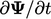in an equation can be discretised as a ﬁnite diﬀerence in time. Timeis expressed in discrete intervals, or steps, of duration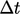.

The Euler scheme calculates the derivative from the ﬁeld at the current time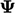and the previous, or old, time level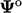by: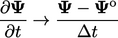(3.21)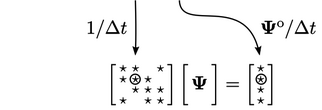Time discretisation thereby contributes to the diagonal (only!) coeﬃcients of the matrix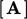and to the source vector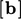.

### Courant number

For a 1D domain in the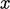-direction, the Courant number is the following dimensionless parameter for each cell of length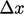: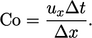(3.22)
The Courant number originates from the solution of 1D advection, e.g. Eq. (2.32 ), with the Euler time scheme of Eq. (3.21 ) and an explicit upwind advection scheme from Sec. 3.10 . For that case, the Courant-Friedrichs-Lewy (CFL) condition12 for convergence is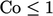across all cells.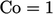corresponds to a ﬂuid particle moving across one cell in one time step, so its relevance to solution convergence is perhaps unsurprising.

In an explicit solution, the convergence limit can reduce further to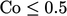or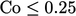, with more accurate schemes for advection. But the ﬁnite volume method is generally implicit so stability can then be maintained with a higher maximum value of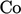. Temporal accuracy is then the important consideration when choosing theof a simulation, both in terms of its mean and maximum value across all cells in the domain.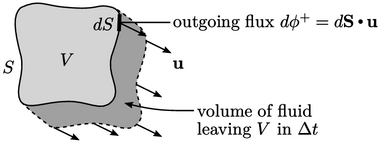It is therefore important to monitor Courant number which needs to be calculated for 3D problems. Explicit, Euler, upwind discretisation of advection can be presented in 3D as follows: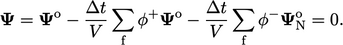(3.23)
Here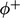are positive ﬂuxes which transports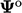out of the cell of interest and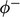are negative ﬂuxes which transports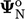from neighbouring cells. Since the CFL condition,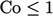, requires that the coeﬃcient of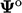cannot be negative, it follows that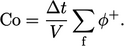(3.24)
This 3D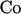represents the volume of ﬂuid leaving the cell in one time step, as a fraction of the cell volume, as shown above.
12after Richard Courant, Kurt Friedrichs and Hans Lewy, Uber die partiellen Diﬀerenzengleichungen der mathematischen Physik, 1928.

Notes on CFD: General Principles - 3.17 Time discretisation